# RD Sharma Solutions for Class 11 Maths Exercise 24.3 Chapter 24 - The Circle

In this exercise, we shall deal with problems related to the concepts of the diameter form of a circle. Students can use this exercise as a model of reference to improve their conceptual knowledge and understand the different methods used to solve the problems. Students can refer to RD Sharma Class 11 Maths Solutions, which are designed by the expert team in Maths to increase students’ confidence, which plays a major role in their board exams. The links to the solutions of this exercise can be accessed in the RD Sharma Class 11 Maths pdf, which is available in the below-mentioned links.

## Download the pdf of RD Sharma Solutions for Class 11 Maths Exercise 24.3 Chapter 24 – The Circle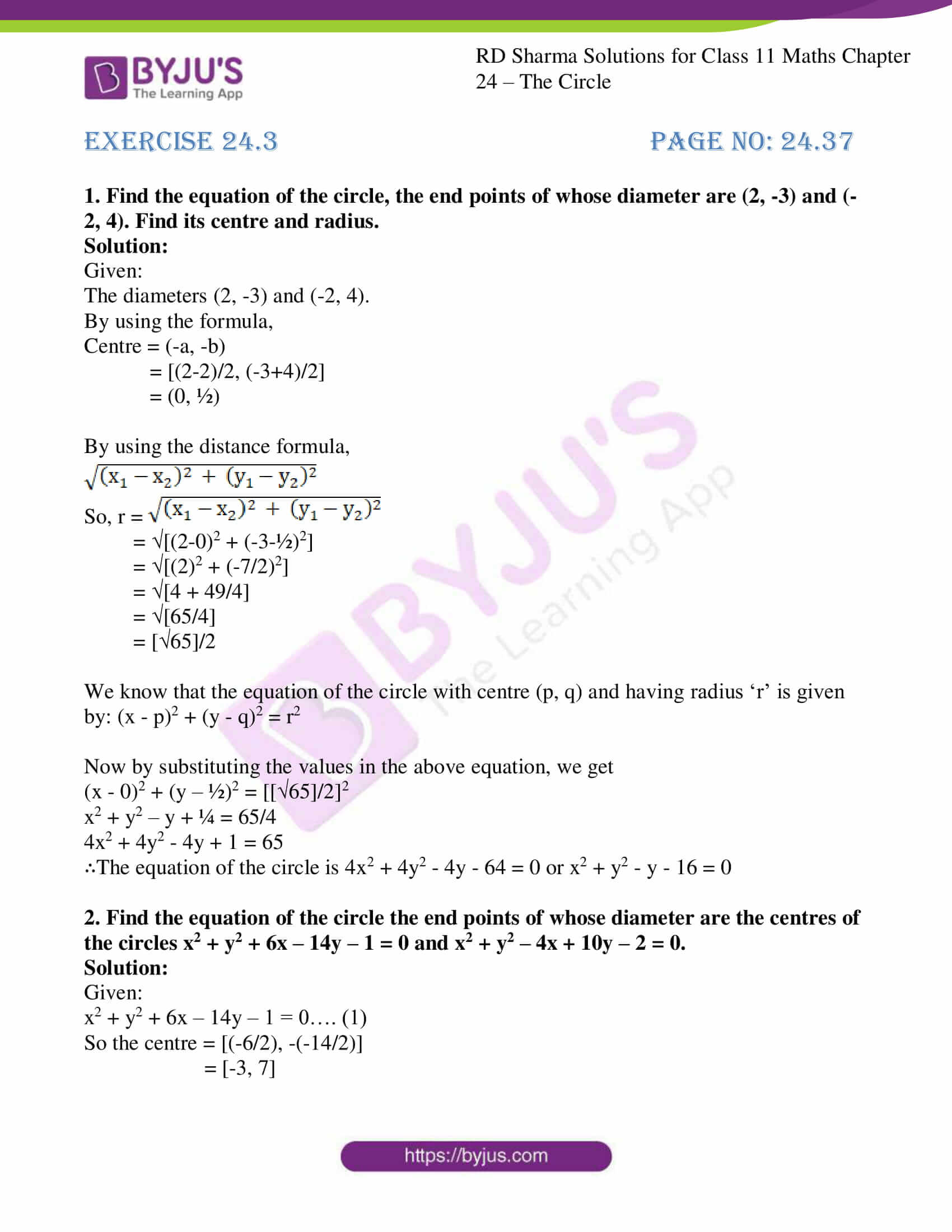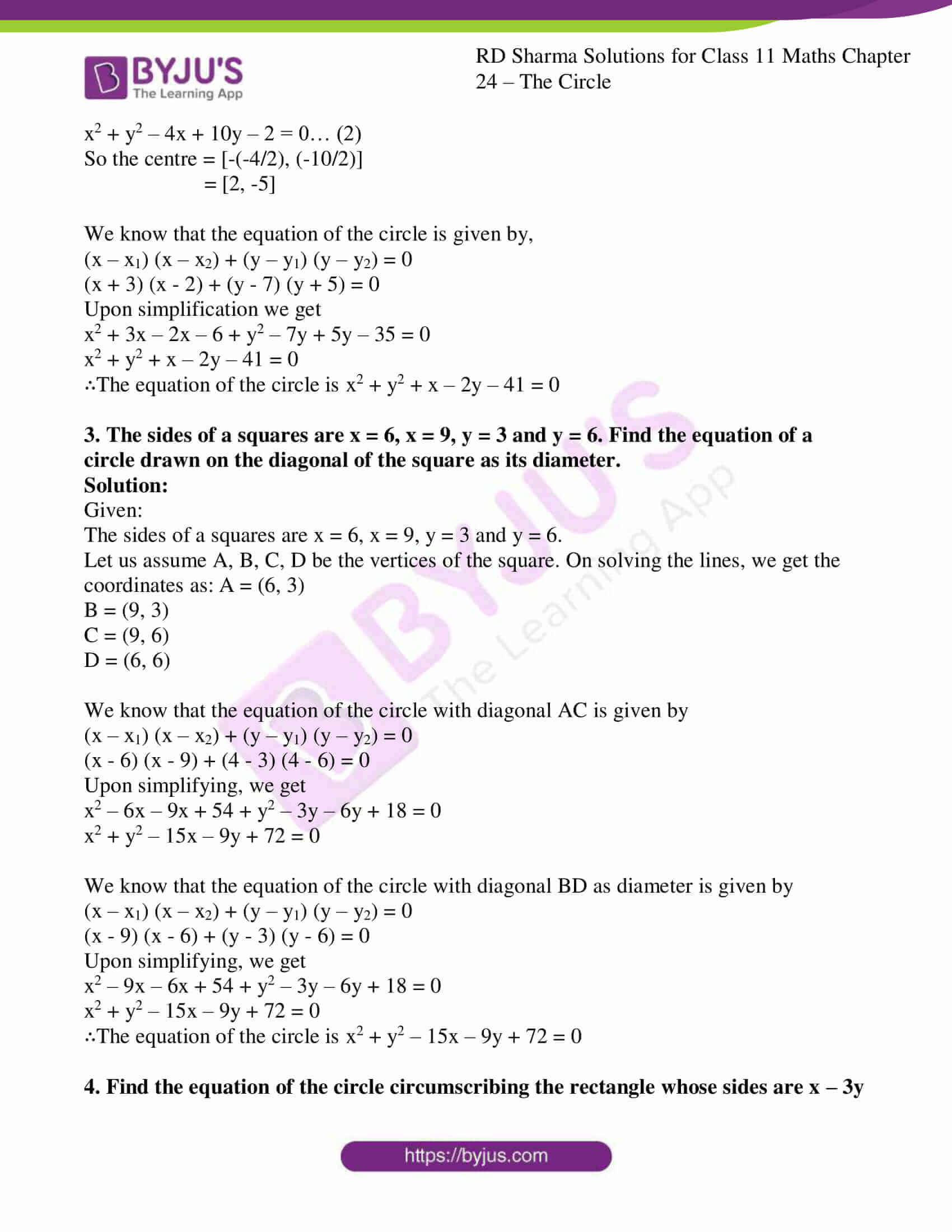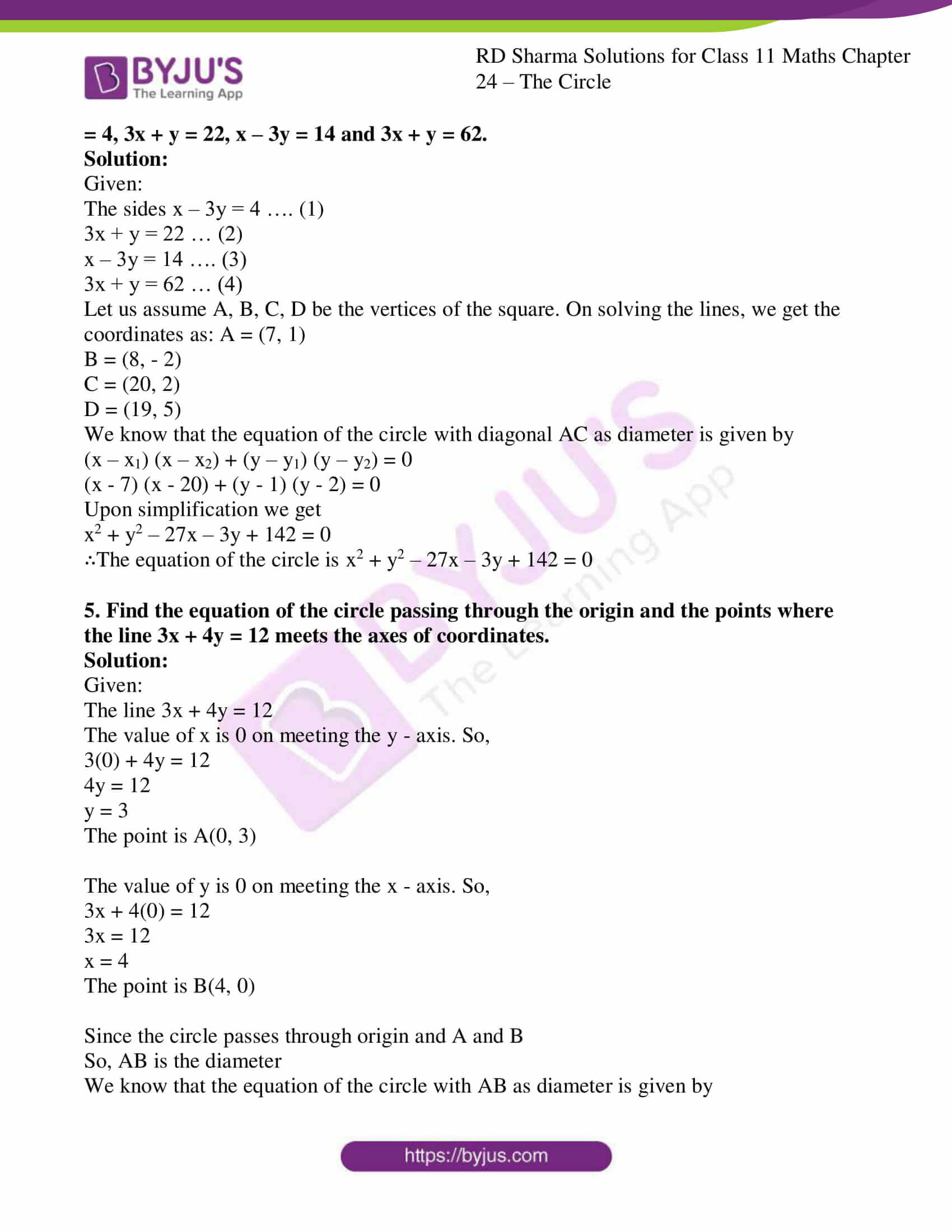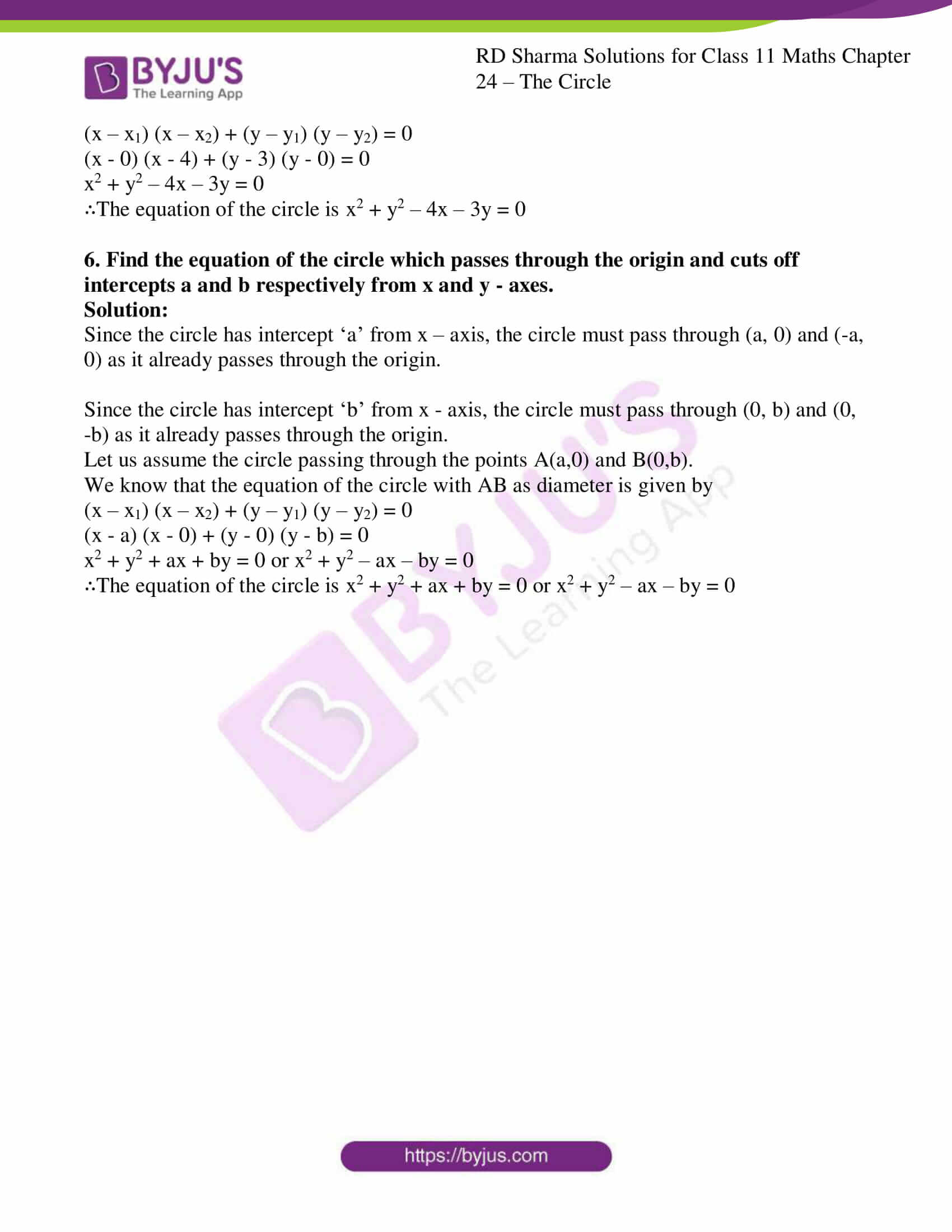### Also, access other exercises of RD Sharma Solutions for Class 11 Maths Chapter 24 – The Circle

Exercise 24.1 Solutions

Exercise 24.2 Solutions

### Access answers to RD Sharma Solutions for Class 11 Maths Exercise 24.3 Chapter 24 – The Circle

1. Find the equation of the circle, the end points of whose diameter are (2, -3) and (-2, 4). Find its centre and radius.

Solution:

Given:

The diameters (2, -3) and (-2, 4).

By using the formula,

Centre = (-a, -b)

= [(2-2)/2, (-3+4)/2]

= (0, ½)

By using the distance formula,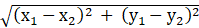So, r == [(2-0)2 + (-3-½)2]

= [(2)2 + (-7/2)2]

= [4 + 49/4]

= [65/4]

= /2

We know that the equation of the circle with centre (p, q) and having radius ‘r’ is given by: (x – p)2 + (y – q)2 = r2

Now by substituting the values in the above equation, we get

(x – 0)2 + (y – ½)2 = [/2]2

x2 + y2 – y + ¼ = 65/4

4x2 + 4y2 – 4y + 1 = 65

∴The equation of the circle is 4x2 + 4y2 – 4y – 64 = 0 or x2 + y2 – y – 16 = 0

2. Find the equation of the circle the end points of whose diameter are the centres of the circles x2 + y2 + 6x – 14y – 1 = 0 and x2 + y2 – 4x + 10y – 2 = 0.

Solution:

Given:

x2 + y2 + 6x – 14y – 1 = 0…. (1)

So the centre = [(-6/2), -(-14/2)]

= [-3, 7]

x2 + y2 – 4x + 10y – 2 = 0… (2)

So the centre = [-(-4/2), (-10/2)]

= [2, -5]

We know that the equation of the circle is given by,

(x – x1) (x – x2) + (y – y1) (y – y2) = 0

(x + 3) (x – 2) + (y – 7) (y + 5) = 0

Upon simplification we get

x2 + 3x – 2x – 6 + y2 – 7y + 5y – 35 = 0

x2 + y2 + x – 2y – 41 = 0

∴The equation of the circle is x2 + y2 + x – 2y – 41 = 0

3. The sides of a squares are x = 6, x = 9, y = 3 and y = 6. Find the equation of a circle drawn on the diagonal of the square as its diameter.

Solution:

Given:

The sides of a squares are x = 6, x = 9, y = 3 and y = 6.

Let us assume A, B, C, D be the vertices of the square. On solving the lines, we get the coordinates as: A = (6, 3)

B = (9, 3)

C = (9, 6)

D = (6, 6)

We know that the equation of the circle with diagonal AC is given by

(x – x1) (x – x2) + (y – y1) (y – y2) = 0

(x – 6) (x – 9) + (4 – 3) (4 – 6) = 0

Upon simplifying, we get

x2 – 6x – 9x + 54 + y2 – 3y – 6y + 18 = 0

x2 + y2 – 15x – 9y + 72 = 0

We know that the equation of the circle with diagonal BD as diameter is given by

(x – x1) (x – x2) + (y – y1) (y – y2) = 0

(x – 9) (x – 6) + (y – 3) (y – 6) = 0

Upon simplifying, we get

x2 – 9x – 6x + 54 + y2 – 3y – 6y + 18 = 0

x2 + y2 – 15x – 9y + 72 = 0

∴The equation of the circle is x2 + y2 – 15x – 9y + 72 = 0

4. Find the equation of the circle circumscribing the rectangle whose sides are x – 3y = 4, 3x + y = 22, x – 3y = 14 and 3x + y = 62.

Solution:

Given:

The sides x – 3y = 4 …. (1)

3x + y = 22 … (2)

x – 3y = 14 …. (3)

3x + y = 62 … (4)

Let us assume A, B, C, D be the vertices of the square. On solving the lines, we get the coordinates as: A = (7, 1)

B = (8, – 2)

C = (20, 2)

D = (19, 5)

We know that the equation of the circle with diagonal AC as diameter is given by

(x – x1) (x – x2) + (y – y1) (y – y2) = 0

(x – 7) (x – 20) + (y – 1) (y – 2) = 0

Upon simplification we get

x2 + y2 – 27x – 3y + 142 = 0

∴The equation of the circle is x2 + y2 – 27x – 3y + 142 = 0

5. Find the equation of the circle passing through the origin and the points where the line 3x + 4y = 12 meets the axes of coordinates.

Solution:

Given:

The line 3x + 4y = 12

The value of x is 0 on meeting the y – axis. So,

3(0) + 4y = 12

4y = 12

y = 3

The point is A(0, 3)

The value of y is 0 on meeting the x – axis. So,

3x + 4(0) = 12

3x = 12

x = 4

The point is B(4, 0)

Since the circle passes through origin and A and B

So, AB is the diameter

We know that the equation of the circle with AB as diameter is given by

(x – x1) (x – x2) + (y – y1) (y – y2) = 0

(x – 0) (x – 4) + (y – 3) (y – 0) = 0

x2 + y2 – 4x – 3y = 0

∴The equation of the circle is x2 + y2 – 4x – 3y = 0

6. Find the equation of the circle which passes through the origin and cuts off intercepts a and b respectively from x and y – axes.

Solution:

Since the circle has intercept ‘a’ from x – axis, the circle must pass through (a, 0) and (-a, 0) as it already passes through the origin.

Since the circle has intercept ‘b’ from x – axis, the circle must pass through (0, b) and (0, -b) as it already passes through the origin.

Let us assume the circle passing through the points A(a,0) and B(0,b).

We know that the equation of the circle with AB as diameter is given by

(x – x1) (x – x2) + (y – y1) (y – y2) = 0

(x – a) (x – 0) + (y – 0) (y – b) = 0

x2 + y2 + ax + by = 0 or x2 + y2 – ax – by = 0

∴The equation of the circle is x2 + y2 + ax + by = 0 or x2 + y2 – ax – by = 0